Download Presentation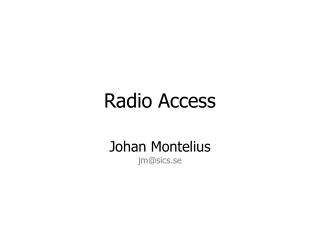# Radio Access - PowerPoint PPT Presentation

Radio Access. Johan Montelius jm@ sics .se. Shannon. C = W x log 2 (1 + S/N). The capacity [C] in bits/s is directly proportional to the available bandwidth [W] and log 2 proportional to the signal to noise ratio [S/N]. bandwidth & power. Attenuation in open space.I am the owner, or an agent authorized to act on behalf of the owner, of the copyrighted work described.
Download PresentationAn Image/Link below is provided (as is) to download presentation

Download Policy: Content on the Website is provided to you AS IS for your information and personal use and may not be sold / licensed / shared on other websites without getting consent from its author.While downloading, if for some reason you are not able to download a presentation, the publisher may have deleted the file from their server.

- - - - - - - - - - - - - - - - - - - - - - - - - - E N D - - - - - - - - - - - - - - - - - - - - - - - - - -
Presentation Transcript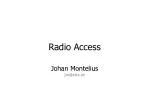Johan Monteliusjm@sics.se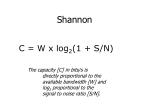Shannon

C = W x log2(1 + S/N)

The capacity [C] in bits/s is directly proportional to the available bandwidth [W] and

log2 proportional to the signal to noise ratio [S/N].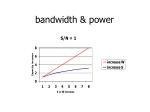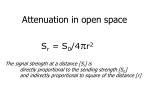Attenuation in open space

Sr = S0/4r2

The signal strength at a distance [Sr] is directly proportional to the sending strength [S0] and indirectly proportional to square of the distance [r]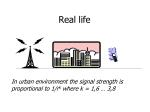Real life

In urban environment the signal strength is proportional to 1/rk where k = 1,6 … 3,8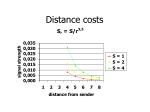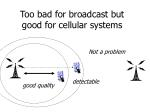Too bad for broadcast but good for cellular systems

Not a problem

detectable

good quality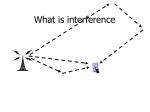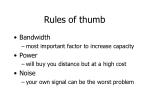Rules of thumb
• Bandwidth
• most important factor to increase capacity
• Power
• will buy you distance but at a high cost
• Noise
• your own signal can be the worst problem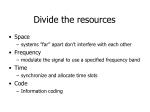Divide the resources
• Space
• systems ”far” apart don’t interfere with each other
• Frequency
• modulate the signal to use a specified frequency band
• Time
• synchronize and allocate time slots
• Code
• Information coding1800

1850

1900

1950

2000

2050

2100

2150

2200

2250

National/International regulations

MSS

ITU

IMT 2000

EU

GSM 1800

UMTS

DECT

MDS

US

PCS

Jp/Ko

IMT 2000

China

GSM 1800

IMT 2000Frequency division
• By modulating a carrier frequency, the radiated power can be limited to a specified frequency range.
• The width of the range is the bandwidth of the carrier.
• A guard band is needed to protect adjacent carriers.Frequency planning

A

B

C

3 cells per site

typically used in urban environmentFrequency planning

4 sites, 3 cells per site

minimum distance

12 carriers needed

A

D

B

E

C

F

A

J

G

H

K

I

LTime division
• Enabled by faster processors.
• A carrier is divided into time slots.
• Each channel is allocated a time slot.
• A guard period is needed between adjacent time slotsA sender must adjust its transmission to meet thetime slot at the receiver. The farther away the earlier you send . The base station will tell you if your late or early.

a

b

B

AWhat is left ?
• when bandwidth is fixed
• and power is limited
• do the best modulation possibleModulation
• frequency modulation
• amplitude modulation
• phase modulation
• combination of above
• … no modulation ?Wireless systems
• Often use a phase modulation
• Could change modulation depending on quality of signal
• Spectral efficiency
• up to 2 bits raw data per Hz under good conditions
• aprx 0,5 to 1 bit user data per Hz
• limited by signal to noise ratioHow do we compare?
• What is the maximum user capacity?
• What is the maximum capacity of a system?
• How many carriers do we have?
• What is the total capacity of a carrier?
• How many carriers can be used at any given point?GSM
• Each duplex carrier is 2x200 KHz wide
• 900
• up 890-915 MHz down 935-960 MHz
• 124 duplex carriers
• 2x25MHz in total
• 1800
• up 1710-1785 MHz down 1805-1880 MHz
• 374 duplex carriers
• 2x75MHz in total !!!!!
• 1900 (in the US)
• up 1850-1910 MHz down 1930-1990
• 2x60MHz in totalGSM
• Time division
• 8 time slots per carrier
• one carrier up one carrier down
• Gaussian Minimum Shift Keying (GMSK)
• user bitrate 9,6 kb/s or 14,4 kb/s per timeslot
• raw bitrate 272 kb/s per carrier
• HSCSD
• Two or more time slotsUp and down

0

1

2

3

4

5

6

7

down

up

The up link is delayed 3 slots in order togive the terminal time to adjust to the new frequency. Time slots 5 and 6 can be used to listen for better frequencies.GPRS
• Dynamically allocate time slots
• normally 1:4 one up, four down
• Data and voice can be combined
• Coding schemes (user data rates)
• CS 1: 9,05 kb/s total 72,4 kb/s
• CS 2: 13,4 kb/s total 107,2 kb/s
• CS 3: 15,6 kb/s total 124,8 kb/s
• CS 4: 21,4 kb/s total 171,2 kb/sEDGE
• Enhanced data rate for GSM Evolution
• Change the modulation to 8-PSK i.e. 3 bits per symbol
• User data rate
• 22,8 kb/s to 69,2 kb/s
• Total of 553 kb/s
• don’t moveUMTS/WCDMA
• Each paired carrier is 2x5MHz
• 1900-1980, 2010-2025, 2110-2170 MHz
• 155 MHz in total
• Unpaired carriers can be used using time-division duplex mode (TDD)
• A typical operator
• Two or three paired, one unpaired
• Up to six operators share the spectrumISM 2.4 GHz
• Industrial, Scientific and Medical
• US 2400 – 24835 83,5MHz in total
• Japan 2400 – 2497 89,7MHz in total
• Open for anyone, no license
• Limitation on power < 0.1W (<1W US)
• Using a spread spectrum technique• Why spread the signal over a wider spectrum?
• more robust, will survive if part of the spectrum is noisy
• will allow other systems to operate in the same environment
• Two techniques
• frequency hopping
• direct sequenceFrequency Hopping
• divide the spectrum into separate carriers
• In ISM, FCC regulated at least 70 carriers
• transmit and hop
• In ISM, FCC regulates < 400 ms
• a code determines where to hop
• how do we synchronize?
• low cost, low power, very robustDirect Sequence
• Increase the bandwidth by sending a pattern, chipping sequence, at a higher bitrate
• sequence can be static or dynamic
• dynamic patterns are used in CDMA
• high bitrate, robustBluetooth 1.1
• Frequency hopping, GFSK modulation
• Gaussian Frequency Shift Key
• 79 carriers of 1 MHz, 1600 hops per s
• Power
• Class 1: 20dBm (100mW) range aprx 100m
• Class 2: 4dBm (2,5 mW) range aprx 10m
• Class 3: 0dbM (1 mW) range aprx 10 cm
• Master & Slave
• Master determines hopping sequence
• Capacity 712 Kb/s per channel802.11b
• DSSS, BPSK (1Mbps) QPSK (11Mbps)
• ISM 2.4
• US 11 carriers
• Europe (except France and Spain) 13 carriers
• Japan 14 carriers
• Carrier
• 22 MHz wide
• can use 3 carriers without overlap!802.11b
• 1 Mb/s using BPSK
• Barker spread sequence of 11 bits
• 2 Mb/s using QPSK
• Barker sequence of 11 bits (22 Mb/s raw data)
• 5,5 and 11 Mb/s
• QPSK, same as for 2Mb/s
• complementary code keying
• 1,375M symbols/s
• each symbol is 8 bits long (11 Mb/s raw data)
• each symbol represents 4 or 8 bits802.11b

11 Mb/s 8 b/symbol 8 chips/symbol 1,375 Msymb/s QPSK

5,5 Mb/s 4 b/symbol 8 chips/symbol 1,375 Msymb/s QPSK

2 Mb/s 1 b/symbol 11 chips/symbol 2 Msymb/s QPSK

1 Mb/s 1 b/symbol 11 chips/symbol 1 Msymb/s BPSKCode division
• Same frequency can be used
• No cell planning
• How do we decode the message?Code division: coding

1

d1

d2

message di

-1

1

code cik

-1

1

Zik= dik * cik

out zik

-1Code division: decoding

1

out zik

-1

1

code cik

-1

S

m

1

di =

zikcik

m

k = 1

1

d1 =

(-1 –1 – 1 –1 – 1 – 1 –1 – 1) = -1

8Code division: multiple senders

Da = -1-1-1-1-1-1-1-1+1+1+1+1+1+1+1+1

Ca = +1+1+1-1+1-1-1-1+1+1+1-1+1-1-1-1

Za = -1-1-1+1-1+1+1+1+1+1+1-1+1-1-1-1

Db = +1+1+1+1+1+1+1+1+1+1+1+1+1+1+1+1

Cb = +1-1+1+1+1-1+1+1+1-1+1+1+1-1+1+1

Zb = +1-1+1+1+1-1+1+1+1-1+1+1+1-1+1+1Code division

Za = -1-1-1+1-1+1+1+1+1+1+1-1+1-1-1-1

Zb = +1-1+1+1+1-1+1+1+1-1+1+1+1-1+1+1

Zab= +0-2+0+2+0+0+2+2+2+0+2+0+2-2+0+0

Zab= +0-2+0+2+0+0+2+2+2+0+2+0+2-2+0+0

Ca = +1+1+1-1+1-1-1-1+1+1+1-1+1-1-1-1

ZCa= +0-2+0-2+0+0-2-2+2+0+2+0+1+2+0+0

Sa= -8/8 = -1 +8/8 = +1Ultra wide band

More than 1.5 GHz or 20% of central frequency

Use low power, low enough to disappear in noise level of other systems

Compensate by using large bandwidth, up to several GHz

Distance is, due to low power, limited < 10 m

UWBShannon revisited
• Shannon’s theorem sets a limit for one receiver listening to one message.
• What happens if we have several channels open, multiple receivers.
• Is there a limitation on capacity in space?WCDMA
• 5 MHz carrier
• QPSK modulation
• 3,84 Mcps chipping rate